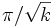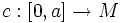Bonnet-Myers theorem

This article describes a result related to the Ricci curvature of a Riemannian manifold

This article describes a result related to the sectional curvature of a Riemannian manifold

This result relates information on curvature to information on topology of a manifold

This article makes a prediction about the universal cover of a manifold based on given data at the level of a:topological manifold

This result is valid in all dimensions

Statement

Ricci curvature version

Here are some equivalent forms:

• Any complete simply connected Riemannian manifold whose Ricci curvature everywhere is bounded below by a positive constant, must be compact
• Any complete Riemannian manifold whose Ricci curvature everywhere is bounded below by a positive constant, must be compact
• If a Riemannian manifold has the property that there exists a positive constant that lower-bounds the

Ricci curvature for all tangent lines at all points, then the manifold is compact with finite fundamental group. This is equivalent to saying that the universal cover of the manifold is compact.

The reason why all these formulations are equivalent is that given any Riemannian manifold, we can consider its universal cover and give the universal cover the pullback metric. In that case, the range of values taken by the Ricci curvature is same for both manifolds.

Sectional curvature version

This makes a stronger assumption, but also gives a stronger result, namely:

Any complete Riemannian manifold whose sectional curvature is everywhere bounded below by a constant$k$, satisfies the property that its diameter (viz, maximum possible distance between two points) is at most$\pi/\sqrt{k}$.

Note that an upper bound on the distance between points implies, for a complete Riemannian manifold, that it is compact.

Facts

Any Einstein metric with positive cosmological constant, for instance, satisfies the hypotheses for the Bonnet-Myers theorem, and hence, any manifold possessing such a metric is compact with finite fundamental group.

Relation with other results

Further information: Cartan-Hadamard theorem The Cartan-Hadamard theorem talks of the analogous statement when the manifold has nonpositive sectional curvature throughout. It says that under that assumption, the universal cover is diffeomorphic to real Euclidean space.

Together, the Cartan-Hadamard theorem and Bonnet-Myers theorem tell us that a complete manifold which has positive curvature bounded from below, cannot be diffeomorphic to a manifold which has negative sectional curvature throughout.

Proof

Proof of the sectional curvature version

The proof hinges on the following result:

If the sectional curvature is bounded below by a positive constant$k$, then any normal geodesic$c:[0,a] \to M$ of length at least$\pi/\sqrt{k}$ contains a conjugate point to its starting point.

Completeness tells us that any two points are joined by a minimal geodesic that does not contain any pair of conjugate points other than possibly the endpoints (by the Hopf-Rinow theorem). Hence the distance between any two points cannot be more than$\pi/\sqrt{k}$.